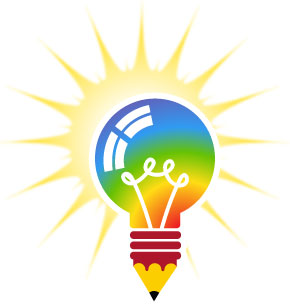Planet Protection 5-6 yrs

Download this Free PDF to help teach about planet protection. It includes crafts and activities and how everyone can do their part to protect the environment!

Does your group need a little motivation to lend a helping hand? Why not search our Nature and Our World categories to find a patch to give out as a reward?

Carly Christenson

This Meeting Plan was researched and written by our intern Carly Christenson. Do you have an idea for an Meeting Plan? Submit your own Idea, Plan, Craft, Camp or Game to smiles@e-patchesandcrests.com and if we post it on our site you will receive 15% off an in-stock order.

Updated Jun 14, 2016Do you have something that you would like to share? We would love to see it! Submit your testimonial, camp plan, meeting idea, craft, recipe, game or any handy information that could be used by others.

Anyone who submits material that we post on our website will receive 15% off an in-stock or custom order.

var brd = document.createElement("DIV"); document.body.insertBefore(brd, document.getElementById("header")); seeds = []; particles = []; const fwkPtcIniV = 0.5; const fwkSedIniV = 0.5; const fwkPtcIniT = 2500; const fwkSedIniT = 1000; const a = 0.0005; const g = 0.0005; const v = 0.3; const cursorXOffset = 5; const cursorYOffset = 0; function newFireworkParticle(x, y, angle) { var fwkPtc = document.createElement("DIV"); fwkPtc.setAttribute('class', 'fireWorkParticle'); fwkPtc.time = fwkPtcIniT; while(angle > 360) angle -= 360; while(angle < 0) angle += 360; fwkPtc.velocity = []; if(angle > 270) { fwkPtc.velocity.x = fwkPtcIniV * Math.sin(angle * Math.PI / 180) * (1 - Math.random() * v); fwkPtc.velocity.y = fwkPtcIniV * Math.cos(angle * Math.PI / 180) * (1 - Math.random() * v); } else if(angle > 180) { fwkPtc.velocity.x = fwkPtcIniV * Math.sin(angle * Math.PI / 180) * (1 - Math.random() * v); fwkPtc.velocity.y = fwkPtcIniV * Math.cos(angle * Math.PI / 180) * (1 - Math.random() * v); } else if(angle > 90) { fwkPtc.velocity.x = fwkPtcIniV * Math.sin(angle * Math.PI / 180) * (1 - Math.random() * v); fwkPtc.velocity.y = fwkPtcIniV * Math.cos(angle * Math.PI / 180) * (1 - Math.random() * v); } else { fwkPtc.velocity.x = fwkPtcIniV * Math.sin(angle * Math.PI / 180) * (1 - Math.random() * v); fwkPtc.velocity.y = fwkPtcIniV * Math.cos(angle * Math.PI / 180) * (1 - Math.random() * v); } fwkPtc.position = []; fwkPtc.position.x = x; fwkPtc.position.y = y; fwkPtc.style.left = fwkPtc.position.x + 'px'; fwkPtc.style.top = fwkPtc.position.y + 'px'; if(particles == null) particles = []; particles.push(fwkPtc); return fwkPtc; } document.querySelector("#sidebar").addEventListener("mouseover", newFireWorkOnClick); document.querySelector("#header").addEventListener("mouseover", newFireWorkOnClick); function newFireWorkOnClick(event) { newFireworkSeed(event.pageX - brd.offsetLeft + cursorXOffset, event.pageY - brd.offsetTop + cursorYOffset); } function newFireworkSeed(x, y) { var fwkSed = document.createElement("DIV"); fwkSed.setAttribute('class', 'fireWorkSeed'); brd.appendChild(fwkSed); fwkSed.time = fwkSedIniT; fwkSed.velocity = []; fwkSed.velocity.x = 0; fwkSed.velocity.y = fwkSedIniV; fwkSed.position = []; fwkSed.position.x = x; fwkSed.position.y = y; fwkSed.style.left = fwkSed.position.x + 'px'; fwkSed.style.top = fwkSed.position.y + 'px'; if(seeds == null) seeds = []; seeds.push(fwkSed); return fwkSed; } function newFireWorkStar(x, y) { var fwkBch = document.createElement("DIV"); fwkBch.setAttribute('class', 'fireWorkBatch'); var a = 0; while(a < 360) { var fwkPtc = newFireworkParticle(x, y, a); fwkBch.appendChild(fwkPtc); a += 5; } brd.appendChild(fwkBch); } var before = Date.now(); var id = setInterval(frame, 5); function frame() { var current = Date.now(); var deltaTime = current - before; before = current; for(i in seeds) { var fwkSed = seeds[i]; fwkSed.time -= deltaTime; if(fwkSed.time > 0) { fwkSed.velocity.x -= fwkSed.velocity.x * a * deltaTime; fwkSed.velocity.y -= g * deltaTime + fwkSed.velocity.y * a * deltaTime; fwkSed.position.x += fwkSed.velocity.x * deltaTime; fwkSed.position.y -= fwkSed.velocity.y * deltaTime; fwkSed.style.left = fwkSed.position.x + 'px'; fwkSed.style.top = fwkSed.position.y + 'px'; } else { newFireWorkStar(fwkSed.position.x, fwkSed.position.y); fwkSed.parentNode.removeChild(fwkSed); seeds.splice(i, 1); } } for(i in particles) { var fwkPtc = particles[i]; fwkPtc.time -= deltaTime; if(fwkPtc.time > 0) { fwkPtc.velocity.x -= fwkPtc.velocity.x * a * deltaTime; fwkPtc.velocity.y -= g * deltaTime + fwkPtc.velocity.y * a * deltaTime; fwkPtc.position.x += fwkPtc.velocity.x * deltaTime; fwkPtc.position.y -= fwkPtc.velocity.y * deltaTime; fwkPtc.style.left = fwkPtc.position.x + 'px'; fwkPtc.style.top = fwkPtc.position.y + 'px'; } else { fwkPtc.parentNode.removeChild(fwkPtc); particles.splice(i, 1); } } } function are_cookies_enabled() { var cookieEnabled = (navigator.cookieEnabled) ? true : false; if (typeof navigator.cookieEnabled == "undefined" && !cookieEnabled) { document.cookie="testcookie"; cookieEnabled = (document.cookie.indexOf("testcookie") != -1) ? true : false; } return (cookieEnabled); } if (!are_cookies_enabled()) { \$('#hello').append('